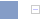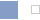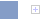# A rough view of the future

### Video

MathML:

$G\left(x,y\right)=\frac{1}{2\pi {\sigma }^{2}}{e}^{-\frac{{x}^{2}+{y}^{2}}{2{\sigma }^{2}}}$